「APIO2009」采油区域 - 最大子矩阵 | Bill Yang's Blog

## 初步想法

•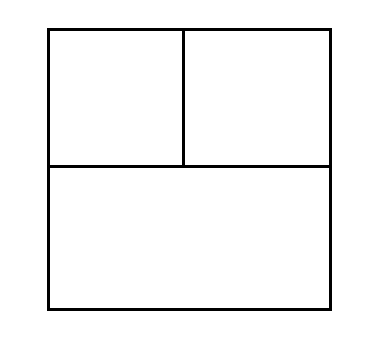•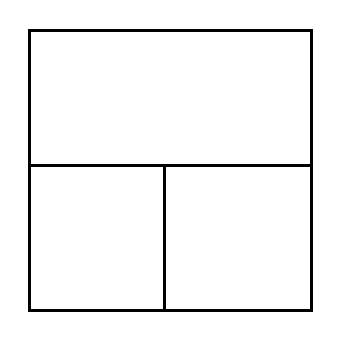•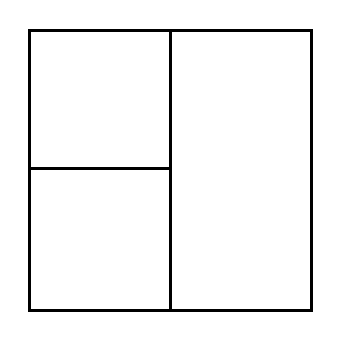•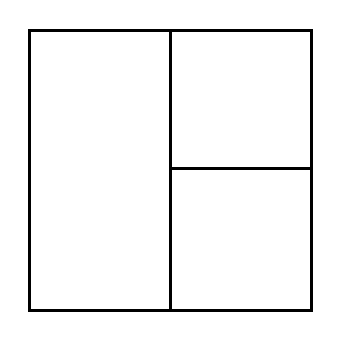••## 具体算法

$fLU[i,j]$：以$(1,1)$为左上角$(i,j)$为右下角的矩阵中的最大子正方形。
$fLD[i,j]$：以$(n,1)$为左下角$(i,j)$为右上角的矩阵中的最大子正方形。
$fRU[i,j]$：以$(1,m)$为右上角$(i,j)$为左下角的矩阵中的最大子正方形。
$fRD[i,j]$：以$(n,m)$为右下角$(i,j)$为左上角的矩阵中的最大子正方形。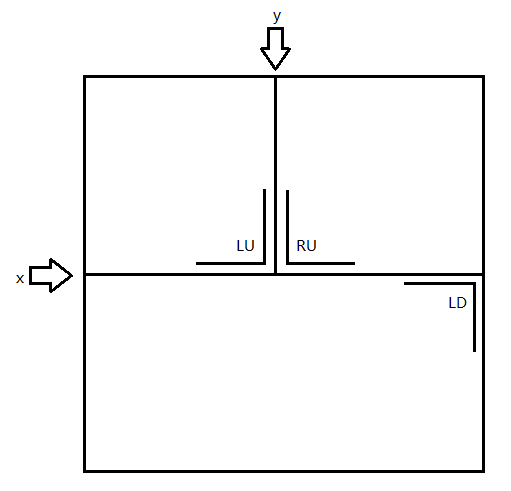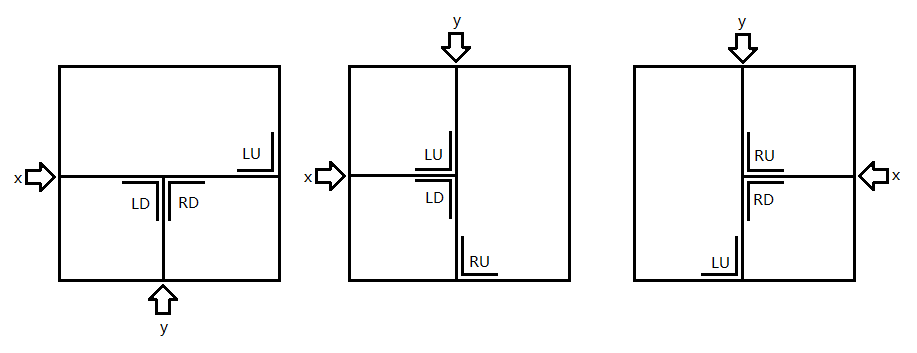②对于后两种情况：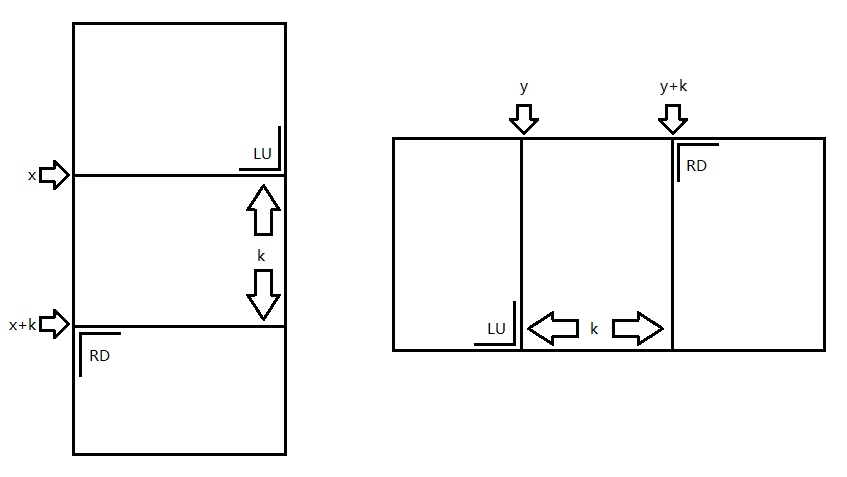0%